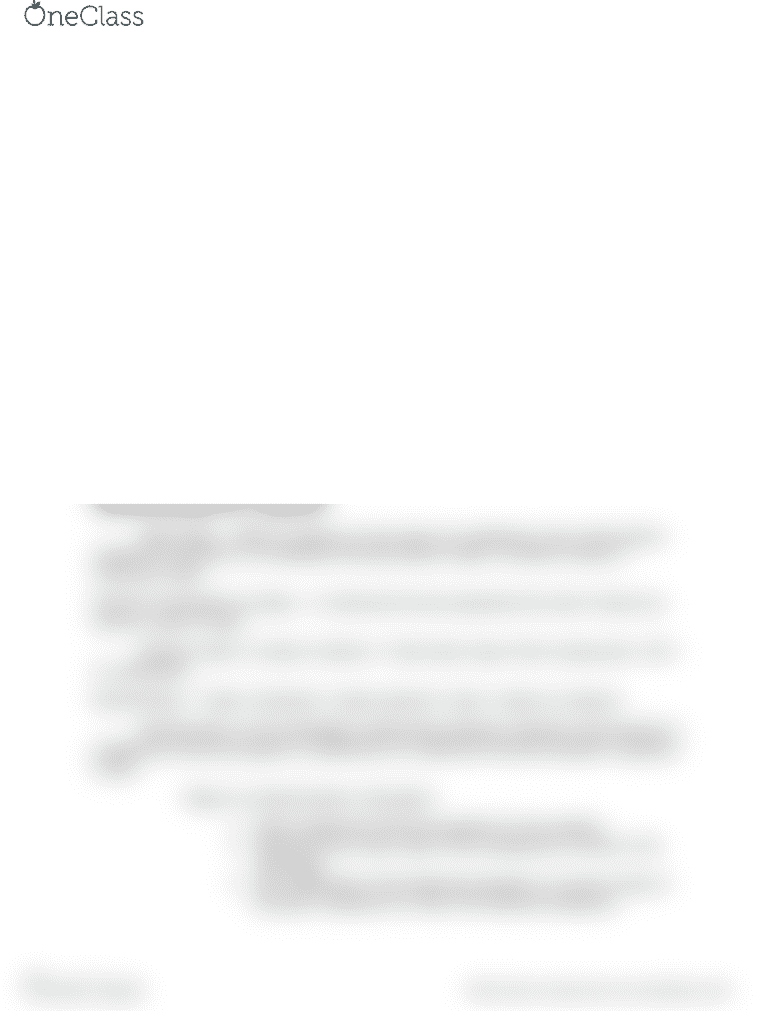# CHEM 1000 Lecture Notes - Lecture 8: Molar Mass, Chemical Equation, Chemical Formula

52 views2 pagesChapter 5 Chemical Accounting
Section 5.1-5.4 Balancing Chemical Equations; Molar Mass; Percent Composition;
Stoichiometry
Chemical Sentences: Equations
Chemical Equations describe a chemical change using symbols and formulas
to represent the elements and compounds involved in a chemical reaction
Carbon reacts with oxygen to form carbon dioxide ( C + O2 = CO2)
Reactants Species present BEFORE the reaction
Products Species present AFTER the reaction
Arrow in a reaction means “yields”
Symbols: (s) = solid (l) = liquid (g) = gas (aq) = aqueous solution (aqueous = water)
Balancing a chemical Equation:
Coefficients numbers used to balance a chemical equation. These numbers are
placed IN FRONT of the molecular formula. NEVER CHANGE THE SUBSCIPTS!!!!
Ex. H2 + N2 → NH3 3H2 + N2 → 2NH3
Determining Mass of a Compound
Molar Mass Mass (in grams) of one mole of a substance; Sum of the atomic
masses for all atoms in a molecular formula (Atomic mass x number of atoms =
molecular mass)
Percent Composition by Mass A chemical formula represents the ratio of atoms as
well as a ratio of mass
[(Atomic mass x number of atoms) / Total molar mass of the compound] x 100 =
% composition
Stoichiometry Greek “stoicheion” means element “metry” means to measure
Stoichiometry is the Quantitative relationship between reactants and products in
a balanced chemical equation (Coefficients of a balanced chemical equation represent
moles)
Steps to a Stoichiometric Calculation:
1. Write a balanced chemical equation for the reaction
2. Determine the molar mass of the substances involved in the
calculation
3. Use coefficients of the balanced equation to convert moles of
the given substance to mole of the desired substance
find more resources at oneclass.com
find more resources at oneclass.com
Unlock document

This preview shows half of the first page of the document.
Unlock all 2 pages and 3 million more documents.

Already have an account? Log in

# Get access

Grade+
\$10 USD/m
Billed \$120 USD annually
Homework Help
Class Notes
Textbook Notes
40 Verified Answers
Study Guides
1 Booster Class
Class+
\$8 USD/m
Billed \$96 USD annually
Homework Help
Class Notes
Textbook Notes
30 Verified Answers
Study Guides
1 Booster Class